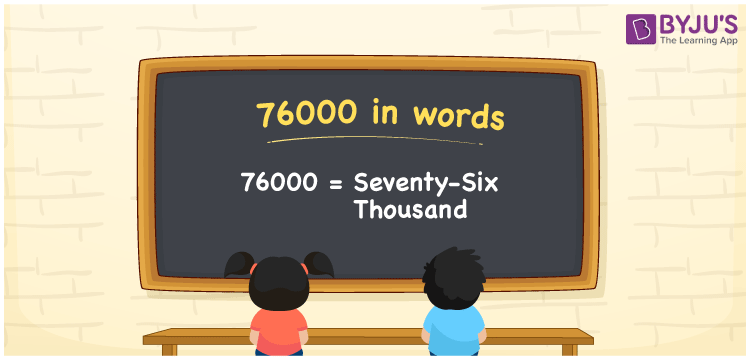# 76000 in words

76000 in words is written as Seventy Six Thousand. In 76000, 7 has a place value of ten thousand and 6 has the place value of thousand. The article on Place Value gives more information. The number 76000 is used in expressions that relate to money, distance, length, population and many more. For example, “The Highest number of Covid cases for a single day were Seventy Six Thousand.”

 76000 in words Seventy Six Thousand Seventy Six Thousand in Numbers 76000

## 76000 in English Words## How to Write 76000 in Words?

We can convert 76000 to words using a place value chart. The number 76000 has 5 digits, so let’s make a chart that shows the place value up to 5 digits.

 Ten thousand Thousands Hundreds Tens Ones 7 6 0 0 0

Thus, we can write the expanded form as:

7 × Ten thousand + 6 × Thousand + 0 × Hundred + 0 × Ten + 0 × One

= 7 × 10000 + 6 × 1000 + 0 × 100 + 0 × 10 + 0 × 1

= 76000

= Seventy Six Thousand.

76000 is the natural number that is succeeded by 75999 and preceded by 76001.

76000 in words – Seventy Six Thousand.

Is 76000 an odd number? – No.

Is 76000 an even number? – Yes.

Is 76000 a perfect square number? – No.

Is 76000 a perfect cube number? – No.

Is 76000 a prime number? – No.

Is 76000 a composite number? – Yes.

## Solved Example

1. Write the number 76000 in expanded form

Solution: 7 x 10000 + 6 x 1000 + 0 x 100 + 0 x 10 + 0 x 1

Or Just 7 x 10000 + 6 x 1000

We can write 76000 = 70000 + 6000 + 0 + 0 + 0

= 7 x 10000 + 6 x 1000 + 0 x 100 + 0 x 10 + 0 x 1

## Frequently Asked Questions on 76000 in words

Q1

### How to write 76000 in words?

76000 in words is written as Seventy Six Thousand.
Q2

### State True or False. 76000 is divisible by 3?

False. 76000 is not divisible by 3.
Q3

### Is 76000 divisible by 10?

Yes. 76000 is divisible by 10.